﻿ 2nd Grade Math Common Core State Standards Worksheets

# 2nd Grade Math Common Core State Standards Worksheets

## Operations & Algebraic Thinking

###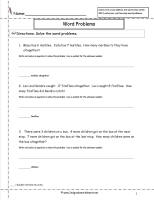CCSS 2.OA.1 Worksheets

Represent and solve problems involving addition and subtraction.

1. Use addition and subtraction within 100 to solve one- and two-step word problems involving situations of adding to, taking from, putting together, taking apart, and comparing, with unknowns in all positions, e.g., by using drawings and equations with a symbol for the unknown number to represent the problem.

###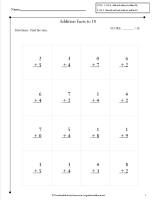CCSS 2.OA.2 Worksheets

2. Fluently add and subtract within 20 using mental strategies. 2 By end of Grade 2, know from memory all sums of two one-digit numbers.

###CCSS 2.OA.3 Worksheets

Work with equal groups of objects to gain foundations for multiplication.

3. Determine whether a group of objects (up to 20) has an odd or even number of members, e.g., by pairing objects or counting them by 2s; write an equation to express an even number as a sum of two equal addends.

###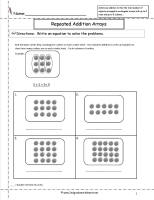CCSS 2.OA.4 Worksheets

Work with equal groups of objects to gain foundations for multiplication.

4. Use addition to find the total number of objects arranged in rectangular arrays with up to 5 rows and up to 5 columns; write an equation to express the total as a sum of equal addends.

## Number and Operations in Base Ten

###CCSS 2.NBT.1 Worksheets

Understand place value.

1. Understand that the three digits of a three-digit number represent amounts of hundreds, tens, and ones; e.g., 706 equals 7 hundreds, 0 tens, and 6 ones. Understand the following as special cases:
a.100 can be thought of as a bundle of ten tens — called a “hundred.”
b.The numbers 100, 200, 300, 400, 500, 600, 700, 800, 900 refer to one, two, three, four, five, six, seven, eight, or nine hundreds (and 0 tens and 0 ones).

###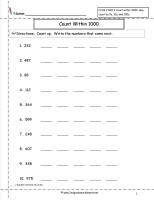CCSS 2.NBT.2 Worksheets

Understand place value.

2. Count within 1000; skip-count by 5s, 10s, and 100s.

###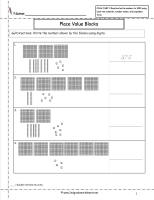CCSS 2.NBT.3 Worksheets

Understand place value.

3. Read and write numbers to 1000 using base-ten numerals, number names, and expanded form.

###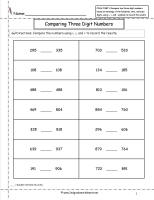CCSS 2.NBT.4 Worksheets

Understand place value.

4. Compare two three-digit numbers based on meanings of the hundreds, tens, and ones digits, using >, =, and < symbols to record the results of comparisons.

###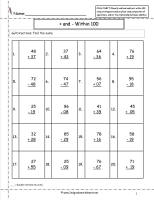CCSS 2.NBT.5 Worksheets

Use place value understanding and properties of operations to add and subtract.

5. Fluently add and subtract within 100 using strategies based on place value, properties of operations, and/or the relationship between addition and subtraction.

###CCSS 2.NBT.6 Worksheets

Use place value understanding and properties of operations to add and subtract.

6. Add up to four two-digit numbers using strategies based on place value and properties of operations.

###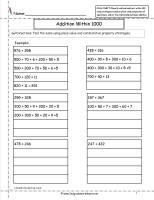CCSS 2.NBT.7 Worksheets

Use place value understanding and properties of operations to add and subtract.

7. Add and subtract within 1000, using concrete models or drawings and strategies based on place value, properties of operations, and/or the relationship between addition and subtraction; relate the strategy to a written method. Understand that in adding or subtracting three-digit numbers, one adds or subtracts hundreds and hundreds, tens and tens, ones and ones; and sometimes it is necessary to compose or decompose tens or hundreds.

###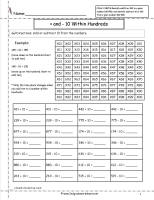CCSS 2.NBT.8 Worksheets

Use place value understanding and properties of operations to add and subtract.

8. Mentally add 10 or 100 to a given number 100–900, and mentally subtract 10 or 100 from a given number 100–900.

###CCSS 2.NBT.9 Worksheets     Construction

Use place value understanding and properties of operations to add and subtract.

9. Explain why addition and subtraction strategies work, using place value and the properties of operations.

## Measurement & Data

###CCSS 2.MD.1 Worksheets

Measure and estimate lengths in standard units.

1. Measure the length of an object by selecting and using appropriate tools such as rulers, yardsticks, meter sticks, and measuring tapes.

###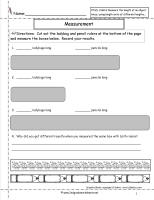CCSS 2.MD.2 Worksheets

Measure and estimate lengths in standard units.

2. Measure the length of an object twice, using length units of different lengths for the two measurements; describe how the two measurements relate to the size of the unit chosen.

###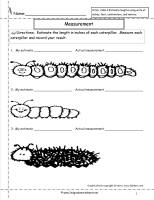CCSS 2.MD.3 Worksheets

Measure and estimate lengths in standard units.

3. Estimate lengths using units of inches, feet, centimeters, and meters.

###CCSS 2.MD.4 Worksheets

Measure and estimate lengths in standard units.

4. Measure to determine how much longer one object is than another, expressing the length difference in terms of a standard length unit.

###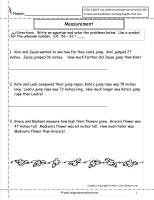CCSS 2.MD.5 Worksheets

Relate addition and subtraction to length.

5. Use addition and subtraction within 100 to solve word problems involving lengths that are given in the same units, e.g., by using drawings (such as drawings of rulers) and equations with a symbol for the unknown number to represent the problem.

###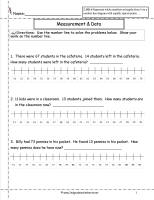CCSS 2.MD.6 Worksheets

Relate addition and subtraction to length.

6. Represent whole numbers as lengths from 0 on a number line diagram with equally spaced points corresponding to the numbers 0, 1, 2, …, and represent whole-number sums and differences within 100 on a number line diagram.

###CCSS 2.MD.7 Worksheets

Work with time and money.

7. Tell and write time from analog and digital clocks to the nearest five minutes, using a.m. and p.m.

###CCSS 2.MD.8 Worksheets

Work with time and money.

8. Solve word problems involving dollar bills, quarters, dimes, nickels, and pennies, using \$ and ¢ symbols appropriately. Example: If you have 2 dimes and 3 pennies, how many cents do you have?

###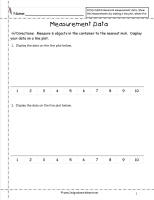CCSS 2.MD.9 Worksheet Represent Data

Common Core State Standards: 2.MD.9
Measurement & Data
Represent and interpret data.

Generate measurement data by measuring lengths of several objects to the nearest whole unit, or by making repeated measurements of the same object. Show the measurements by making a line plot, where the horizontal scale is marked off in whole-number units.

###CCSS 2.MD.10 Worksheets

Represent and interpret data.

10. Draw a picture graph and a bar graph (with single-unit scale) to represent a data set with up to four categories. Solve simple put-together, take-apart, and compare problems 1 using information presented in a bar graph.

## Geometry

###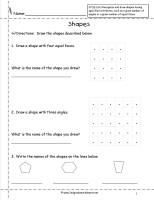CCSS 2.G.1 Shapes Worksheet

Common Core State Standards: 2.G.1
Geometry
Reason with shapes and attributes.

Recognize and draw shapes having specified attributes, such as a given number of angles or a given number of equal faces. Identify triangles quadrilaterals, pentagons, hexagons, and cubes.

###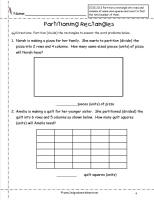CCSS 2.G.2 Partition Rectangles Worksheet

Common Core State Standards: 2.G.2
Geometry
Reason with shapes and attributes.

Partition a rectangle into rows and columns of same-size squares and count to find the total number of them.

###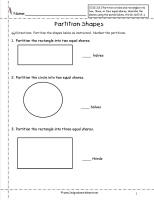CCSS 2.G.3 Partition Shapes Worksheet

Common Core State Standards: 2.G.3
Geometry
Reason with shapes and attributes

Partition circles and rectangles into two, three, or four equal shares, describe the shares using the words halves, thirds, half of, a third of, etc., and describe the whole as two halves, three thirds, four fourths. Recognize that equal shares of identical wholes need not have the same shape